## Math 480 Course Notes -- May 23, 1996

### Crash Course on Probability, Part II

Two interesting random variables came up in response to homework problems from preceding lecture. the first could be called a geometric random variable (with parameter r). It is defined on the sample space {0,1,2,3,...} of non-negative integers and, given a fixed value of r between 0 and 1, has probability function given by(Actually in class we only considered the case where r=1/2, the p(k) from class is p(k-1) here, but the general case isn't any harder). The geometric series formula: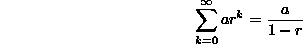shows that p(k) is a probability function. We can compute the expectation of a random variable X having a geometric distribution with parameter r as follows (the trick is reminiscent of what we used in the last lecture on the Poisson distribution):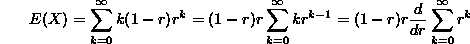Another distribution given in response to the homework assignment was again infinite discrete, defined on the positive integers {1,2,3,...} by the function p(k)=. This is a probability function based on the famous formula due to Euler: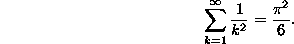But an interesting thing about this distribution concerns its expectation: Because the harmonic series diverges to infinity, we have that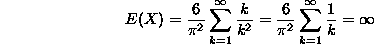So the expectation of this random variable is infinite (Can you interpret this in terms of an experiment whose outcome is a random variable with this distribution?)

### Moments, variance, etc.

The preceding example leads more or less naturally to a discussion of the "moments" of a random variable. Before a discussion of these, we note that if X is a random variable (discrete or continuous), it is possible to define related random variables by taking various functions of X, sayor sin(X) or whatever.

#### Functions of a random variable

If Y=f(X) for some function f, then the probability of Y being in some set A is defined to be the probability of X being in the set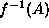.

As an example, consider an exponentially distributed random variable X with parameter=1. Let Y=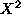. Since X can only be positive, the probability that Y is in the interval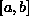is the same as the probability that X is in the interval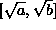.

We can calculate the probability density function p(y) of Y by recalling that the probability that Y is in the interval [0,y] (actually) is the integral of p(y) from 0 to y. In other words, p(y) is the integral of the function h(y), where h(y) = the probability that Y is in the interval [0,y]. But h(y) is the same as the probability that X is in the interval [0,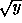]. We calculate: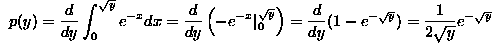There are two ways to calculate the expectation of Y. The first is obvious: we can integrate yp(y). The other is to make the change of variables y=in this integral, which will yield (Check this!) that the expectation of Y=isMore generally, if f(x) is any function, then the expectation of the function f(X) of the random variable X is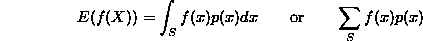where p(x) is the probability density function of X if X is continuous, or the probability function of X if X is discrete.

Now we can talk about the moments of a random variable. The rth moment of X is defined to be the expected value of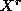. In particular the first moment of X is its expectation. If s>r, then having an sth moment is a more restrictive condition than having and rth one (this is a convergence issue as x approaches infinity, since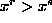for large values of x.

A more useful set of moments is called the set of central moments. These are defined to be the rth moments of the variable X-E(X). In particular, the second moment of X-E(X) is called the variance of X (it is a crude measure of the extent to which the distribution of X is spread out from its expectation value). It is a useful exercise to work out that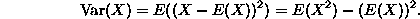As an example, we compute the variance of the uniform and exponential distributions:

• 1. Uniform discrete: If X has a discrete uniform distribution on the interval [a,b], then recall that E(X)=(b+a)/2. We calculate Var(X) as follows: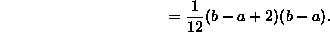• 2. Uniform continuous: If X has a continuous uniform distribution on the interval [a,b], then its variance is calculated as follows: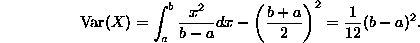• 3. Exponential with parameter: If X is exponentially distributed with parameter, recall that E(X)=1/. Thus: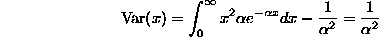The variance decreases asincreases; this agrees with intuition gained from the graphs of exponential distributions shown last time.

### Bivariate distributions:

It is often the case that a random experiment yields several measurements (we can think of the collection of measurements as a random vector) -- one simple example would be the numbers on the top of two thrown dice. When there are several numbers like this, it is common to consider each as a random variable in its own right, and to form the joint probability density (or joint probability) function p(x,y,z,...). For example, in the case of two dice, X and Y are discrete random variables each with sample space S={1,2,3,4,5,6}, and p(x,y)=1/36 for each (x,y) in the cartesian product S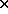S. More generally, we can consider discrete probability functions p(x,y) on sets of the form ST, where X ranges over S and Y over T. Any function p(x,y) such that p(x,y) is between 0 and 1 for all (x,y) and such that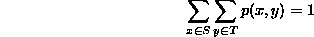defines a (joint) probability function on ST.

For continuous functions, the idea is the same. p(x,y) is the joint pdf of X and Y if p(x,y) is non-negative and satisfiesWe will use the following example of a joint pdf throughout the next section:

X and Y will be random variables that can take on values (x,y) in the triangle with vertices (0,0), (2,0) and (2,2). The joint pdf of X and Y will be given by p(x,y)=1/(2x) if (x,y) is in the triangle and 0 otherwise. Here is a graph of this pdf over its domain of definition:To see that this is a probability density function, we need to integrate p(x,y) over the triangle and get 1: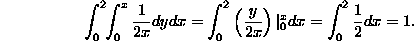More next time on Marginal distributions and Conditional Probability.

### Homework problems:

1. For each of the examples of random variables you gave in the last homework, calculate the variance, if it exists.

2. Calculate the variance of the binomial, Poisson and normal distributions. The answer for the normal distribution is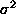.

3. Let X be any random variable with finite second moment. Consider the function f(a) defined as follows: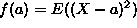. Show that the minimum value of f occurs when a=E(X).

4. Fill in the details of the calculation of the variance of the uniform and exponential distributions. Also, prove that5. Let X be a random variable with the standard normal distribution. find the mean and variance of each of the following: |X|,and.

6. Let X be the sine of an angle in radians chosen uniformly from the interval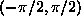. Find the pdf of X, its mean and its variance.

Dennis DeTurck
Wed May 29 17:40:10 EDT 1996